# WORKDAY Function

Returns a date that is N working days in the future or in past

## What is the WORKDAY Function?

The WORKDAY function is categorized under Excel Date and Time functions. It will provide us with a date that is N working days in the future or in the past. We can also use the WORKDAY function to add or subtract days from a given date.

In financial analysis, WORKDAY will be useful in calculating the number of workdays worked by employees, the due date of invoices, the delivery time expected for an order, or a debtor aging schedule.

### Formula

=WORKDAY(start_date, days, [holidays])

The function uses the following arguments:

1. Start_date (required function) – This is a date that represents the start date.
2. Days (It is a required function) – The number of workdays to be added to start_date.
3. [holidays] (optional argument) – This specifies an array of dates that are not included as workdays. The list can be either a range of cells that contain the dates or an array constant of the serial numbers that represent the dates.

In entering dates, Microsoft recommends that the start_date and [holidays] arguments should be entered as either:

1. References to cells containing dates
2. Dates returned from formulas

If we input date arguments as text, there is a risk that Excel may misinterpret them, depending on the date system or date interpretation settings on your computer.

The WORKDAY function will exclude weekends, that is, Saturday and Sunday. If we need to customize which days of the week are considered weekend days, use the more robust WORKDAY.INTL function.

### How to use the WORKDAY Function in Excel?

The WORKDAY function was introduced in Excel 2007 and is available in all subsequent Excel versions. In earlier versions, we need to enable the Analysis ToolPak.

To understand the uses of the function, let’s consider a few examples:

#### Example 1

Suppose we wish to generate a series of dates that are workdays, which is from Monday to Friday.

We need to generate from 01/15/2018. The formula to use will be: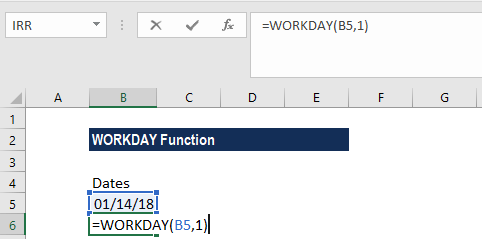We inserted the start date in A2 and then used the formula in A3. Later, we need to simply drag the formula down. We get the results below: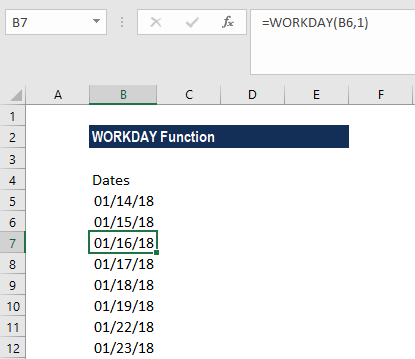MS Excel will solve this formula by using the WORKDAY function to return the next workday. WORKDAY is programmed to skip forward as needed to the next business day.

As we can see in the above results, it skipped weekends (January 20 and 21) and provided January 22 as a workday. If we want to take into account holidays, we need to add the optional argument to the WORKDAY function for holidays.

#### Example 2

The WORKDAY function can be useful in getting the next business day or next working day.  Suppose we are given the following data: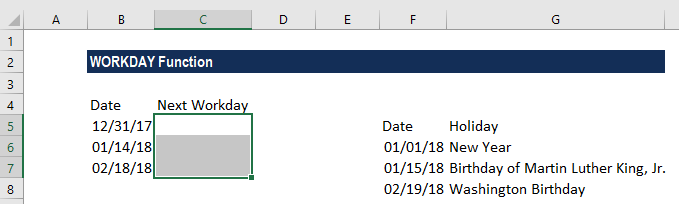The formula used was: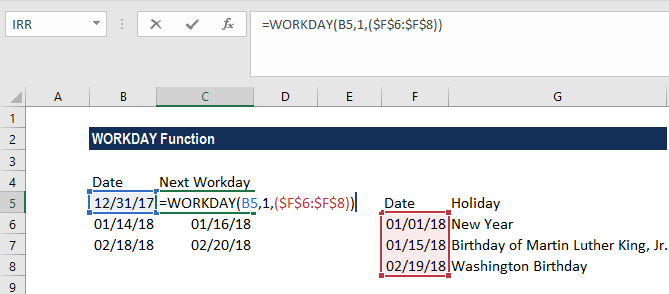Here, we provided Holidays as the named range holidays (E3:E5), so holidays are taken into account as well. Excel would be concerned only with dates and not with which holiday it is. That is, it is not concerned with the actual name of the holiday.

We get the results below: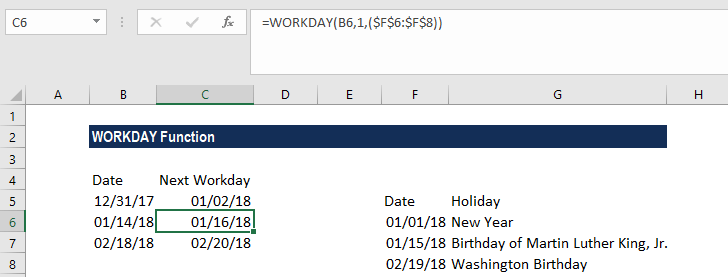### A few notes about the WORKDAY Function

1. If we wish to customize weekends, we need to use WORKDAY.INTL.
2. #NUM error – Occurs when the start_date provided and the given days argument result in an invalid date.
3. If the days provided are not an integer, they are truncated.
4. MS Excel stores dates as sequential serial numbers so they can be used in calculations. By default, January 1, 1900 is serial number 1, and January 1, 2018 is serial number 43101, because it is 43,101 days after January 1, 1900.
5. #VALUE error – Occurs when:
1. The given start_date or any of the values provided in the [holidays] array are not valid dates.
2. The given days argument is non-numeric.

Thanks for reading CFI’s guide to the Excel WORKDAY function. By taking the time to learn and master these functions, you’ll significantly speed up your financial modeling. To learn more, check out these additional CFI resources:

• Excel Functions for Finance